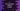# 3 different C programs to find the remainder without modulo### C program to find remainder without using modulo operator:

In this tutorial, we will learn how to find the remainder without using the modulo operator, `%` in C programming language. We will learn three different ways to find the remainder in `C`.

### Method 1: By subtracting the divisor from the number:

1. First of all, read the values of the number and divisor as entered by the user.
2. Keep subtracting the divisor from the number and assign this value to the number until the number becomes smaller than the divisor.
3. If the number becomes smaller than the divisor, it should be the required remainder.
4. Print out the result.

#### Example:

For example, if the number is `10` and the divisor is `3`.

• First, we will calculate `10 - 3`, which is `7` and this value is assigned to the `number`.
• We need to repeat the same step. It will be `7 - 3`, i.e. `4`. In the next step, it will be `4 - 3 = 1`. Since `1` is smaller than `3`, it is the remainder.

Let’s take a look into the program :

#### C program :

``````#include <stdio.h>

int main()
{
// 1
int no, divisor, remainder;

// 2
printf("Enter the number: ");
scanf("%d", &no);

// 3
printf("Enter the divisor: ");
scanf("%d", &divisor);

// 4
while (no >= divisor)
{
no = no - divisor;
}

// 5
remainder = no;

// 6
printf("The remainder is %d", remainder);

return 0;
}``````

#### Explanation :

The commented numbers in the above program denote the step numbers below:

1. Create three integer variables to assign the value of the number(`no`), divisor(`divisor`), and the remainder(`remainder`).
2. Ask the user to enter the number and assign it to the variable `no`.
3. Ask the user to enter the divisor and assign it to the variable `divisor`.
4. Run one `while` loop. Check if `no` is greater than `divisor` or not and if it is, assign the value of `no - divisor` to `no`. Run this loop until the value of `no` is greater than or equal to `divisor`.
5. Assign the final value of `no` to the variable `remainder`.
6. Finally, print out the value of the `remainder`.

#### Sample Output :

``````Enter the number: 12
Enter the divisor: 4
The remainder is 0

Enter the number: 555
Enter the divisor: 4
The remainder is 3``````

### Method 2: Find the remainder with a loop:

We can run a loop and multiply the divisor with a multiplier value. It will keep running until the product is smaller than or equal to the number and on step, we will increase the multiplier value by 1.

The loop will stop if the product is greater than the number. At the end of the loop, we can subtract the divisor value from the final product and subtract it from the number to get the remainder.

• For example, if the number is 100 and the divisor is 3, the product will be 102, since the loop will stop when the product of a number with 3 becomes greater than 100.
• So, the remainder is `number - (product - divisor)` or `100 - (102 - 3)` or `1`.

Let’s write down the complete program:

``````#include <stdio.h>

int getRemainder(int no, int divisor)
{
int multiplier = 1;
int product = 0;

while (product <= no)
{
product = divisor * multiplier;
multiplier++;
}

return no - (product - divisor);
}

int main()
{
int no, divisor, remainder;

printf("Enter the number: ");
scanf("%d", &no);

printf("Enter the divisor: ");
scanf("%d", &divisor);

remainder = getRemainder(no, divisor);

printf("The remainder is %d", remainder);

return 0;
}``````

It will give similar results.

### Method 3: With division and multiplication:

If we subtract the value of `divisor * (no / divisor)` from the number, it will always give the remainder. For example:

``````#include <stdio.h>

int getRemainder(int no, int divisor)
{
return no - divisor * (no / divisor);
}

int main()
{
int no, divisor, remainder;

printf("Enter the number : ");
scanf("%d", &no);

printf("Enter the divisor : ");
scanf("%d", &divisor);

remainder = getRemainder(no, divisor);

printf("The remainder is %d ", remainder);

return 0;
}``````

The `getRemainder` method will provide the same output. For example,
• If the value of `no` is 100 and the `divisor` is 3,
• `no / divisor` is 33
• `divisor * (no / divisor)` is 99
• `no - divisor * (no / divisor)` is 1.
• If the value of `no` is 100 and the `divisor` is 7,
• `no / divisor` is 14
• `divisor * (no / divisor)` is 98
• `no - divisor * (no / divisor)` is 2.